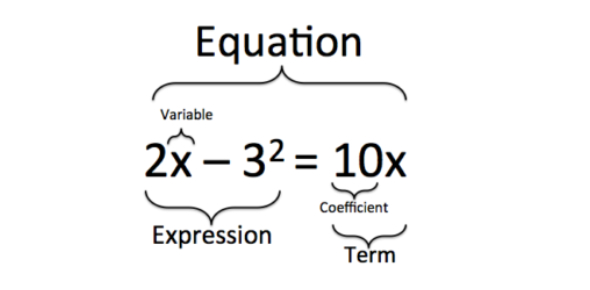# Math: Are You Able To Solve The Equation Question? Quiz

20 Questions | Attempts: 136
ShareSettings.

• 1.
1. Solve the following system of equations by elimination or substitution:      y = -3 - x             2x - y = -3
• A.

(3,2)

• B.

(-1,5)

• C.

(-2,-1)

• D.

(0,-3)

• 2.
Solve the following system of equations by graphing or substitution:   y = x +2      y = 2x -1
• A.

(3,1)

• B.

(3,5)

• C.

(2,-5)

• D.

(1,3)

• 3.
Which best describes the effect on the graph of    y = 4x + 8  if the y-intercept is changed to -3?
• A.

The slope decreases

• B.

The new line passes through the origin.

• C.

The y-intercept decreases.

• D.

The slope increases.

• 4.
• A.

This is a linear funtion

• B.

• C.

This is an exponential growth function

• D.

This is an exponential decay function

• 5.
Which best describes the effect on the graph of   y = 4x + 8 if the slope is changed to 5?
• A.

The slope increases.

• B.

The slope decreases.

• C.

The new line passes through the origin.

• D.

The y-intercept decreases

• 6.
Which of the following is the graph of  y = (1/2)x + 4
• A.
• B.
• C.
• D.
• 7.
Which equation best describes the relationship between x and y ?  (-4,-11)  (-1,-2) (2,7) (5,16)
• A.

Y = (1/3)x + 1

• B.

Y = (1/3)x - 1

• C.

Y = 3x + 1

• D.

Y = 3x - 1

• 8.
The equation c = 0.75t  represents c, the total cost of t tickets on a bus.  Which table contains values that fit this equation? t 1 2 3 4 c \$0.75 \$1.00 \$1.25 \$1.50    A         t 1 2 3 4 c \$0.75 \$1.50 \$2.25 \$3.00    B     t 1 2 3 4 c \$1.75 \$2.50 \$3.25 \$4.00   C     D t 1 2 3 4 c \$1.75 \$2.75 \$3.75 \$4.75
• A.

A

• B.

B

• C.

C

• D.

D

• 9.
What does the domain refer to in a set of data?
• A.

A place where the data lives.

• B.

All the positive values in the data set.

• C.

All possible x values.

• D.

All possible y values.

• 10.
What does the range refer to in a set of data?
• A.

All the negative values in the data set.

• B.

A place where the deer and the antelope play.

• C.

The average of the data.

• D.

All possible y values.

• 11.
The graph of y = x^2 - 2   is shown below. If the graph is shifted up 6 units, which of the following will be the function for the new graph?
• A.

Y = x^2

• B.

Y = 6x^2

• C.

Y = x^2 - 10

• D.

Y = x^2 + 4

• 12.
If the original function y = x^2 is changed to y = x^2 - 2  what has happened to the graph?
• A.

A. It was reflected over the x axis.

• B.

B. It was shifted down 2 spaces.

• C.

C. It was shifted to the left 2 spaces.

• D.

D. It was shifted to the right 2 spaces.

• 13.
If the original function y = x^2 is changed to y = 3x^2 , what has happened to the graph?
• A.

It has been shifted up 3 spaces.

• B.

It’s been vertically stretched (narrower) by a factor of 3.

• C.

It has been shifted down 3 spaces.

• D.

It has been flattened (wider) by a factor of 3.

• 14.
What are the zeros (roots, solutions) of  y = x^2 - 5x +6?
• A.

-2 and -3

• B.

-5 and -1

• C.

5 and 1

• D.

2 and 3

• 15.
Tommy earned \$450 last week. If 15% is taken out for taxes, how much is his paycheck?
• A.

D. \$6750.00

• B.

C. \$382.50

• C.

B. \$435.00

• D.

A. \$67.50

• 16.
I want to purchase a home that is listed at \$140,000. I plan on putting 15% of the list price down on my mortgage. How much is my down payment?
• A.

\$933,333

• B.

\$119,000

• C.

\$21,000

• D.

\$14,000

• 17.
If you make \$40,000 a year, and you pay \$700 a month for rent, what percent of your yearly salary do you spend on rent?
• A.

21%

• B.

31%

• C.

25%

• D.

20%

• 18.
Which of the following is NOT an advantage to having a credit card?
• A.

They are convenient and easy to use

• B.

They allow you to spend more money at once

• C.

It’s easier to order things online

• D.

The danger of losing cash is minimized

## Related TopicsBack to top
×

Wait!
Here's an interesting quiz for you.